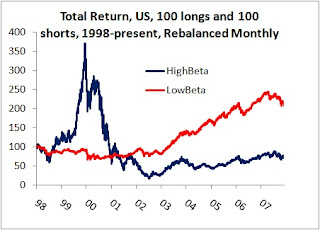## Thursday, March 13, 2008

### The Importance of Geometric AveragingThe graph above shows the total return to my High and Low Beta indices. Every 6 months, starting in 1998, I took the top 1500 market cap companies, and put the companies with the highest 100 betas in the 'high beta' portfolio, and those with the lowest 100 betas in the 'low beta' portfolio.

Over the long run, the high beta portfolio is a stinker. The summary stats over 10 years (up to last weekend) are listed below.Note that the arithmetic average return is about the same for both portfolios, but the geometric much lower for the more highly volatile high beta portfolio. This is because the difference between the geometric and arithmetic averages is the variance of returns divided by 2. The trick is to keep everything in the same time units, so that if we are looking at annualized returns, we need the annualized variance. Note that the adjustment for the high beta portfolio is about 10%, which in turn matches what is actually calculated when I merely used the following methods:

Arithmetic Annual Average: Average(C2:C2498)* 252
Geometric Annual Average: {=PRODUCT(C2:C2498+1)^(1/10)-1}

For a long/short strategy, an assumption as to when one rebalances is key.

For many futures trades, you can assume daily rebalancing, because transaction costs are so low, and people try to keep their first and second order exposures square up (e.g., flat delta and vega), but for equity strategies, a long holding period is appropriate, and this has drastic affects on the returns, especially for highly volatile strategies (note the effect on the 13% vol strategy was pretty minor).